aCute A premium quality meshing software.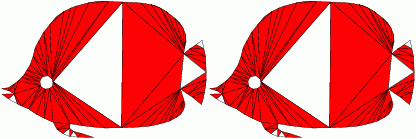Implemented and licensed at the University of Florida, Gainesville
Partially funded by an NSF CAREER Award and an NSF Regular Grant
Contact: Alper Ungor, CISE Department, University of Florida, Gainesville, FL, 32611 ungoratcisedotufldotedu

# Quality Comparison

Here, we demonstrate some features of aCute in generating premium quality triangulations. First, the termination problem is illustrated, where the previous Delaunay refinement algorithms result in an infinite loop when the constraint minimum angle around 34 degrees. Then, sample output quality triangulations generated by aCute are given. Next, sample output triangulations generated by aCute with no small no large angles are shown, where aCute combines minimum and maximum angle constraints at the same time. Also, our results on uniform triangulations are shown, where we use minimum triangle area as the additional constraint to generate uniform quality triangulations.

### Termination Problem

Shewchuks experimental study revealed that the Delaunay refinement algorithm in practice works better than its theoretical guarantee [She96]:

Ruppert [Rup93] proves that this procedure halts for angle constraint of up to 20.7 degrees. In practice, the algorithm generally halts with an angle constraint of 33.8 degrees, but often fails to terminate given an angle constraint of 33.9 degrees. It would be interesting to discover why the cut-off falls there.

The iterative refinement process could introduce smaller and smaller features and may not terminate which is referred to the termination problem. This behavior can be shown as follows: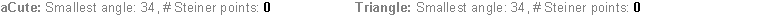### Minimum Angle Quality

#### Triangle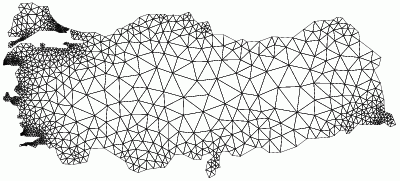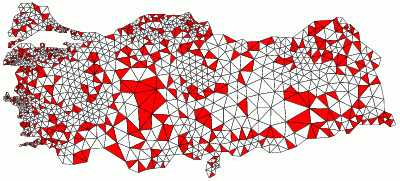Smallest angle: 41 degrees

Smallest angle: 33 degrees
Does not terminate for smallest angle > 34.1 degrees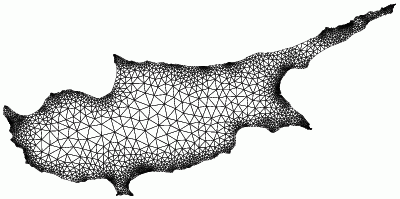Smallest angle: 41 degrees

Smallest angle: 32 degrees
Does not terminate for smallest angle > 33.9 degrees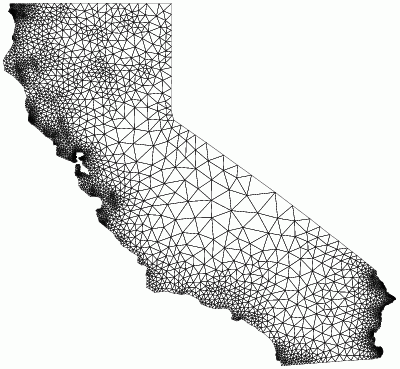Smallest angle: 42 degrees

Smallest angle: 33 degrees
Does not terminate for smallest angle > 34 degrees

### Minimum Angle and Maximum Angle Quality

#### Triangle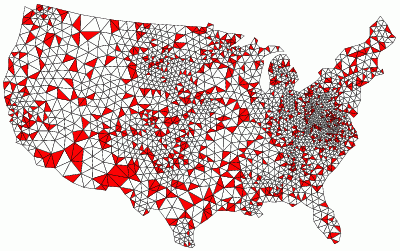Smallest angle: 35 degrees
Largest angle: 85 degrees

Smallest angle: 34.012 degrees
Largest angle: 111.68 degrees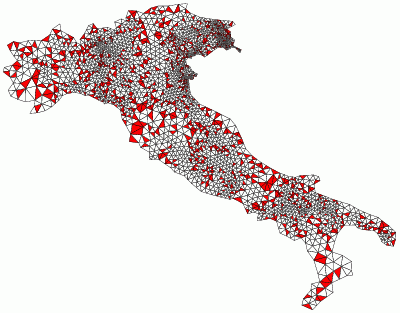Smallest angle: 40 degrees
Largest angle: 88 degrees

Smallest angle: 34.01 degrees
Largest angle: 111.98 degrees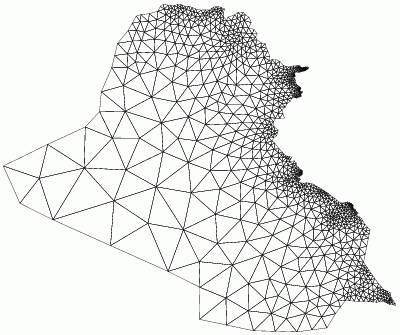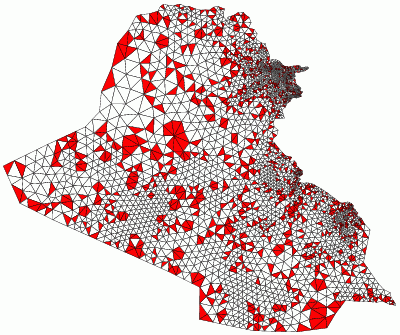Smallest angle: 40 degrees
Largest angle: 88 degrees

Smallest angle: 34.003 degrees
Largest angle: 111.95 degrees

### Premium Quality Uniform Triangulations

#### TriangleSmallest angle: 41.5 degrees
Minimum area = 150
Shortest edge = 1

Smallest angle: 33 degrees
Minimum area = 150
Shortest edge = 1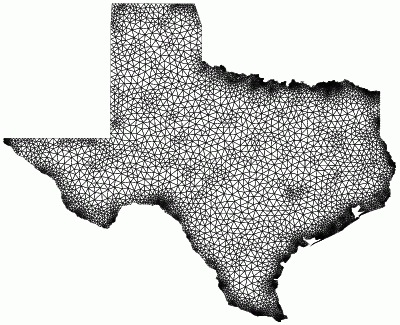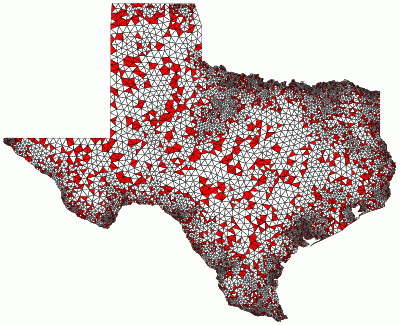Smallest angle: 41.5 degrees
Minimum area = 325
Shortest edge = 1

Smallest angle: 33 degrees
Minimum area = 325
Shortest edge = 1Smallest angle: 38 degrees, Largest angle: 88 degrees
Minimum area = 0.003, Shortest edge = 0.0036674

Smallest angle: 32.017 degrees, Largest angle: 115.36 degrees
Minimum area = 0.003, Shortest edge = 0.0036674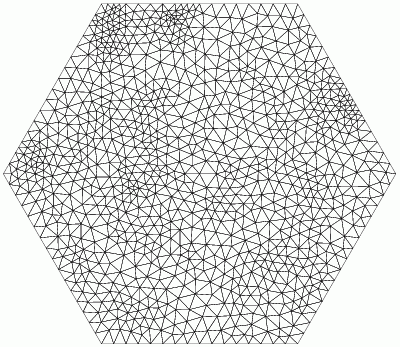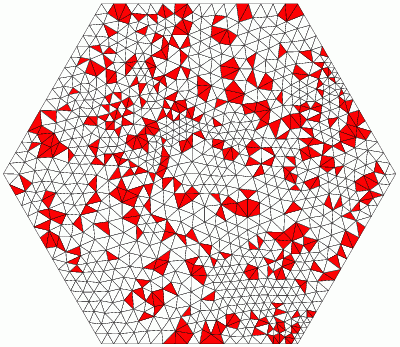Smallest angle: 40 degrees, Largest angle: 86 degrees
Minimum area = 0.05, Shortest edge = 0.3

Smallest angle: 34 degrees, Largest angle: 111.87 degrees
Minimum area = 0.05, Shortest edge = 0.3